Math Quiz For Everybody
Solution to brainteaser on Facebook : there are 4 sisters
Question 1 of 10
What is the remainder when we divide 30 by 4?
Question 2 of 10
What is the distance around a shape called?
Question 3 of 10
What is the perimeter of a circle also called?
Question 4 of 10
What is 3/4 in decimal form?
Question 5 of 10
What does the 8 mean in the number 582?
Question 6 of 10
What are the factors in this problem: 2 x 8 = 16?
Question 7 of 10
What is 3291 rounded to the nearest 1000?
Question 8 of 10
In the fraction 3/4, which number is the denominator?
Question 9 of 10
What does the symbol ">" mean in math?
Question 10 of 10
A mixed number contains what two elements?General Trivia Quiz
Let's see how smart you really are!General trivia quiz
10 questions in mixed themesTrivia quiz for people who are
10 mixed questions to have fun with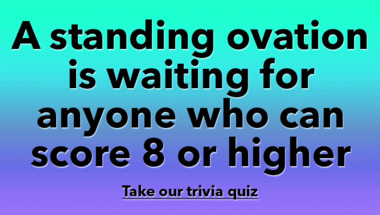Super fun trivia quiz
Only members of the intellectual elite will s..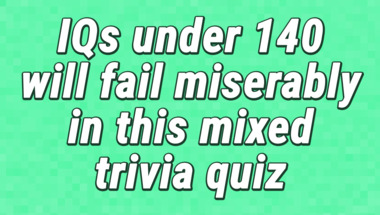Mixed knowledge questions
Trivia quiz for experts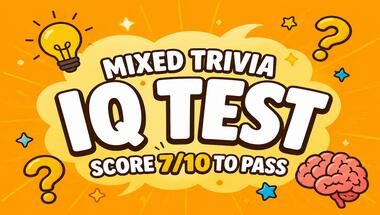General Trivia Quiz
10 mixed up questions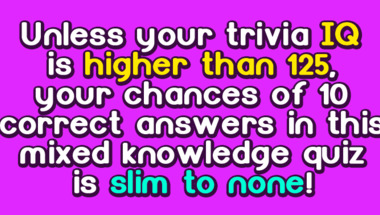History Quiz
10 questions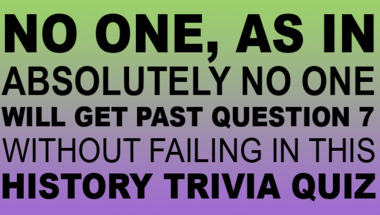Hard trivia quiz
Your goal is to score 5/10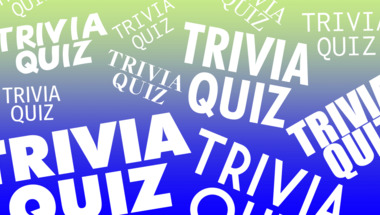Trivia IQ challenge for everyo
10 questions to measure your knowledge1970s Song Quiz
10 songs to guessSuper hard trivia quiz
10 mixed questions for youQuiz : The meaning of words
10 questions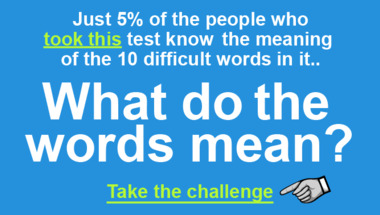General Trivia Quiz
High IQ questions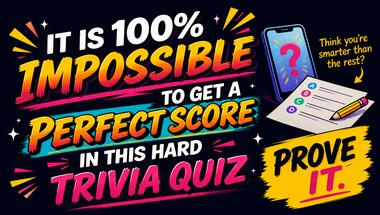General Trivia Quiz
10 mixed questions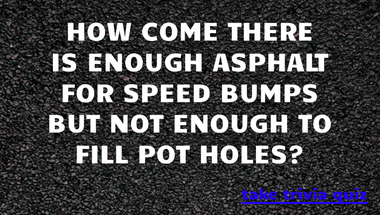World History Quiz
Did you pay attention in school?Copyright 2022 - Wicked Media ApS